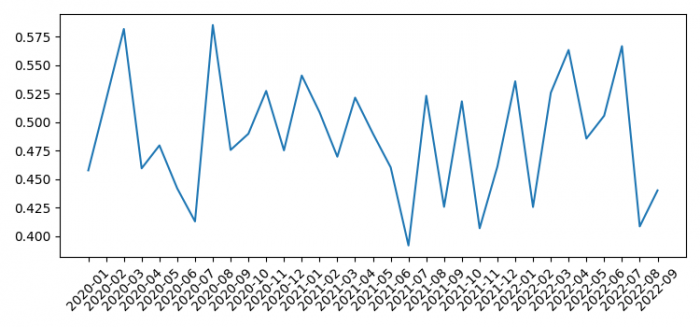# How to plot a Pandas multi-index dataFrame with all xticks (Matplotlib)?

To plot a Pandas multi-index data frame with all xticks, we can take the following steps −

• Set the figure size and adjust the padding between and around the subplots.
• Create index value with 1000 smaples data.
• Make a one-dimensional ndarray with axis labels.
• Get the mean value of the series.
• Plot g dataframe.
• Set the ticks and ticklabel on the current axes
• To display the figure, use show() method.

## Example

import numpy as np
import matplotlib.pyplot as plt
import pandas as pd

plt.rcParams["figure.figsize"] = [7.50, 3.50]
plt.rcParams["figure.autolayout"] = True

idx = pd.date_range("2020-01-01", periods=1000)
val = np.random.rand(1000)
s = pd.Series(val, idx)

g = s.groupby([s.index.year, s.index.month]).mean()

ax = g.plot()
ax.set_xticks(range(len(g)))
ax.set_xticklabels(["%s-%02d" % item for item in g.index.tolist()],
rotation=45, ha='center')

plt.show()

## Output